# MCAT Physical : Universal Gravitation

## Example Questions

### Example Question #1 : Universal Gravitation

Two objects with masses of M and m, sit r distance apart.  What will be the effect on the gravitational force between them if the masses are changed to 2M and 3m?

1. It will increase 36-fold.
2. It will increase 6-fold.
3. It will increase 3-fold.
4. It will not change unless r is changed.
5. None of the above.

1

4

2

5

3

2

Explanation:

Choice 2 is correct.  The formula for gravitational force, G, is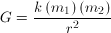, where k is a constant. The effect of doubling one mass and trebling the other is multiplicative,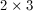, so the answer is six-fold. The question attempts to confuse the respondent by forcing them to recall that the element of the equation which is squared is distance between objects.

### Example Question #1 : Universal Gravitation

An object has a mass of 50kg and a weight of 500N when it is resting on the surface of the Earth. If it is moved to a height equal to three times the Earth’s radius, what is the object’s new weight?

130N

30N

300N

60N

30N

Explanation:

You should be familiar with the following equation for the force of gravity.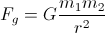To solve this problem, recognize that weight (Fg) is proportional to the inverse square of the radius. When the object was a distance of r (Earth’s radius) it had a weight of 500N. Now, the object is at a distance of 4r (radius of Earth plus the 3r distance that the object is moved to). With the proportion described above, we can see that the force is decreased by a factor of (4)2.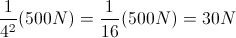### Example Question #1 : Understanding Gravity And Gravitational Forces

The moon's distance from the center of the Earth was decreased by a multiple of three. How would this affect the gravitational force of the Earth on the moon?

It would decrease by a factor of nine

It would increase by a factor of three

The gravitational force would not be affected, because the moon's mass is the same

It would increase by a factor of nine

It would increase by a factor of nine

Explanation:

The law of gravitation is written as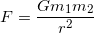, with G being equal to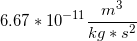.

Since the radius of the two masses acting on each other is squared, and is found in the denominator, a decrease in the radius by a multiple of three will cause a nine-fold increase in the gravitational force.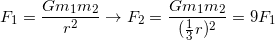### Example Question #18 : Forces

A body with massis situated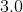meters from a second body with a mass of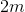. What will be the effect on gravitational attraction of moving one body so that it is only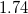meters from the other body?

It will increase by a factor ofIt will increase by a factor ofIt will decrease by a factor ofIt will increase by a factor ofIt will decrease by a factor ofIt will increase by a factor ofExplanation:

Gravity is essentially a property of mass, and the force of gravitational attraction between two bodies is given by the formula: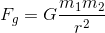In our scenario, the masses remain the same, and of course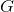is a constant, so the only thing that changes is the denominator.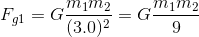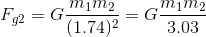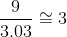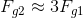### Example Question #1 : Universal Gravitation

Using Newton's Law of Universal Gravitation equation, which of the following expressions is equal to the local gravitational acceleration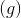on Earth?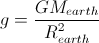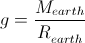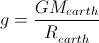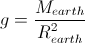Explanation:

On earth,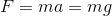.

The law of universal gravitation is equal to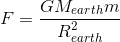.

We can set these equations equal to one another and isolate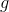by dividing both sides by, the mass of an object on Earth.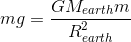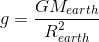Using the mass of the Earth, the radius of the Earth, and the gravitational constant,, we get a value of approximately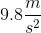if we solve for.

### Example Question #2 : Universal Gravitation

Which of the following must be true for a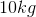block to be considered weightless?

The block is being constantly accelerated upward atThe block is being constantly accelerated downward atThe block is being constantly accelerated downward at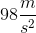The object can never be considered weightless

The block is being constantly accelerated downward atThe block is being constantly accelerated downward atExplanation:

One way an object can be considered "weightless," is if it is accelerating downward at the same rate that it would be if it were free falling. This is why objects in an elevator whose cable had been cut would be "weightless." Therefore the answer is just for the block to be accelerating in the same magnitude and direction as the acceleration due to gravity, which is### Example Question #2 : Universal Gravitation

Which of the following changes would increase a satellite's orbital speed?

Decrease the mass of the satellite

Decrease the mass of the Earth

Increase the mass of the satellite

Explanation:

We know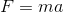, and the force of gravity is: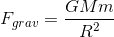Also, the equation for uniform circular motion, such as a satellite in orbit is: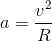Set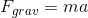and substitute the acceleration due to circular motion into the equation. Solve for velocity.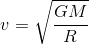This indicates that the only variables that affect the orbital speed are orbital radius and the mass of the Earth.